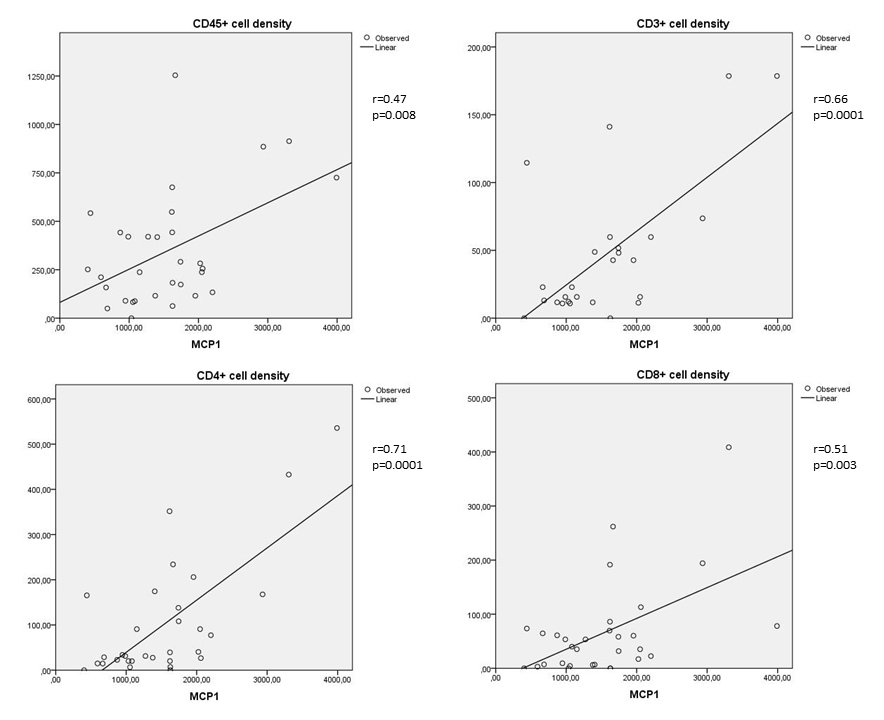Figure 1 of Urbančič, Mol Vis 2020; 26:472-482.

Figure 1. Correlations between MCP-1 and the numerical areal densities of lymphocytes (Spearman's coefficent r). Upper left: correlation between MCP-1 and the numerical areal density of CD45+ cells (r = 0.47; p = 0.008); upper right: correlation between MCP-1 and the numerical areal density of CD3+ cells (r = 0.66; p = 0.0001); lower left: correlation between MCP-1 and the numerical areal density of CD4+ cells (r = 0.71; p = 0.0001); lower right: correlation between MCP-1 and numerical areal density of CD8+ cells (r = 0.51; p = 0.003). Numerical areal density, NA.